Question: Trilogy Innovations(CodeNation) Coding Round, 5th March | Find the Evil Monsters | Minimize Travel Tax | Expected Path Length - III | Three Subarrays
10
Entering edit mode

## Question 1

### Find the Evil Monsters

Given an array A of N elements representing the monsters and an array B of Q elements representing the heros.
The i-th type of monster is represented by A[i], A[i] and A[i] which means a monster of the i-th type is present at each integer co-ordinate from A[i] to A[i] and having a strength of A[i].
The i-th type of hero is represented by B[i] and B[i] which means a hero of strength B[i] will appear at the integer point B[i] after i seconds. When the i-th hero appears it will destroy each and every monster present at B[i] and having a strength less than B[i].
For each i you have to determine the number of monsters left after the i-th hero has completed their task.

Problem Constraints

• 1 <= N <= 105
• 1 <= Q <= 105
• 1 <= A[i] <= A[i] <= 105
• 1 <= B[i] <= 105
• 1 <= A[i] <= 109
• 1 <= B[i] <= 109

## Question 2

### Minimize Travel Tax

There are A cities connected by A-1 bi-directional roads such that all the cities are connected.
Roads are given by array 2D array B where ith road connects B[i] city to B[i].

You go on C trips where on the i-th trip you travel from C[i] city to C[i] city. All the trips are independent of each other.
You have to pay a tax on D[i] on entering or leaving the i-th city. If you pay the tax while entering then you don't need to pay at the time of leaving.

You can choose some non-adjacent cities and make their tax half.
What can be the minimum sum of taxes you pay for all the C trips? Since the answer can be large, return the remainder after dividing it by 109+7.

Problem Constraints

• 2 <= A <= 105
• 1 <= B[i], B[i] <= A
• 1 <= C <= 105
• 1 <= C[i],C[i] <= A
• C[i] != C[i]
• 1 <= D[i] <= 105
• D= A and D[i] % 2 = 0

## Question 3

### Expected Path Length - III

You have A natural number from 1 to A, each occuring exactly once. Now, you choose any of the A (each number being equally likely) numbers and do the following procedures:
1. Initialize a variable step = 0.
2. Let X be the current number you have.
3. Randomly choose any divisor of X (each divisor being equally likely). Let it be Y, now replace X by X/Y. Also, increment step by 1.
4. If X is not equal to 1, repeat from 2nd step.

Let expected value of step be represented in the form of a irreducible fraction x/y. Return xy-1 mod (109+7), where y-1 is the modulo multiplicative inverse of y modulo (109 + 7).

NOTE: You only have to consider positive values of Y.

Problem Constraints

• 1 <= A <= 105

## Question 4

### Three Subarrays

You are given an array A of N integers. You will have to pick 3 subarrays from the array l1, l2 and I3. The subarray I1 must be a prefix and I3 must be a suffux. The minimum length of each of these subarrays must be 1. An element of the array cannot be present in more than 1 of these subarrays.
Find the maximum sum of all the elements in each of these three subarrays. Since the sum can be large, return the positive remainder after dividing the sum with 109 +7.

Problem Constraints

• 3 <= N <= 105
• -109 <= A[i] <= 109

Input Format

• First argument A is an array of integer.

Output Format

• Return an integer
7
Entering edit mode

# Problem 4 Solution

## Topics Involved / Prerequisites

• Dynamic Programming

## Overview

We can solve this problem easily using Dynamic Programming. Consider any choice of the placement of the three subarrays as shown in the figure.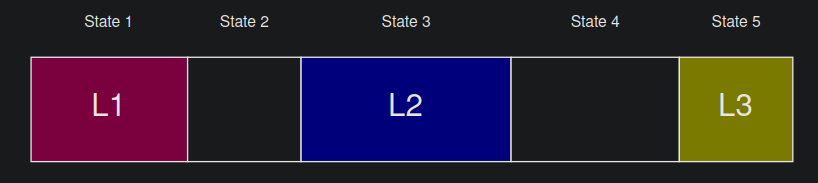We can notice that choosing the three subarrays divides the array into 5 parts (denoted as State 1 through State 5) in the figure. Now State 2 and State 4 can be of length 0 but as specified in the problem statement, there should atleast be 1 element in State 1, 3 and State 5 each. We can use this visualisation to come up with a dynamic programming solution and the corresponding transitions.

## Approach

Let dp[i][j] define the maximum sum of elements by considering the first i elements of the array and being currently in state j (1 <= j <= 5).

The base case is dp = A

We can use the following tranisitions :

``````//keep the same state
dp[i][j] = max(dp[i][j], dp[i-1][j]+(j&1)*A[i]);

//move to next state
if(j!=5) dp[i][j+1] = max(dp[i][j+1], dp[i-1][j] + ((j+1)&1)*A[i]);

//skip state 2 or state 4 to directly jump 2 states ahead as they can be empty
if((j%2 == 1) and j+2<=5) dp[i][j+2] = max(dp[i][j+2], dp[i-1][j] + ((j+2)&1)*A[i]);``````

Here we make use of the fact that we only add A[i] to our sum if we are state 1, 3 and 5 (that is the state no is odd) to simplify the implementation.

Thus we can solve the given problem in a time and space complexity of O(N) using the explained dynamic programming solution.

Entering edit mode
2

Hey, I was wandering why we can't just use kadane to find max subarray l2 then choose max pref and stuff according to l2.
something like this?
`res = max(res,(pdp[i-1]+sdp[j+1]+sm ));`

6
Entering edit mode

# Problem 2 Solution

## Topics Involved / Prerequisites

• Lowest Common Ancestor
• Prefix Sums
• Dynamic Programming

## Overview

If we could determine the number of trips in which we pay the tax for some node, we can estimate the contribution of the node in the sum. We can do this using the idea of prefix sums on tree. Now that we can reduce the taxes for nodes that are not adjacent, we can use dynamic programming to solve this subproblem and calculate the required answer.

## Approach

Prefix Sums on Trees

Suppose that we want to add 1 to the path from node 5 to node 6. Now we add 1 each to node 5 and node 6. Also we subtract 1 from the LCA of node 5 and 6 and also the parent of LCA(if it exists). We then do a post order dfs summing up the updates to obtain the final tree state.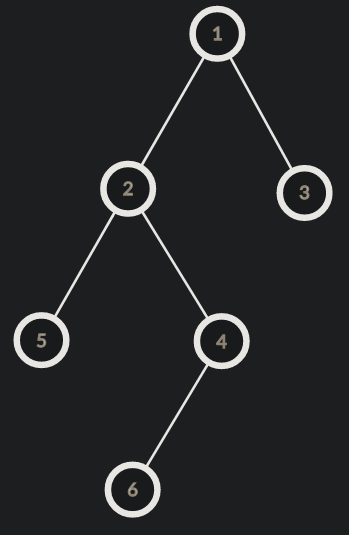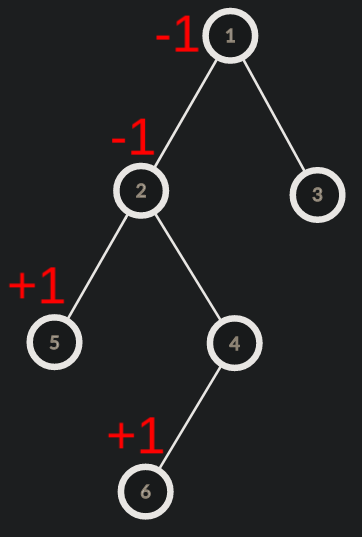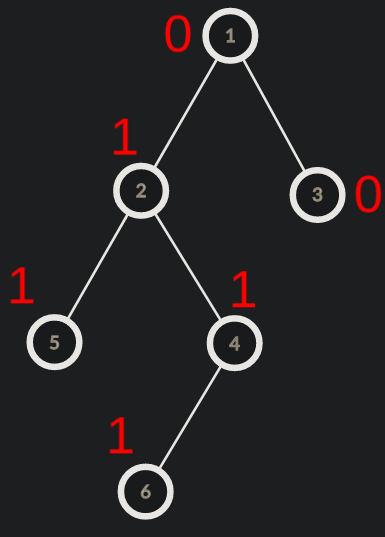Now to perform these updates efficiently, we need to be able to find LCA of two nodes efficiently. This can be done using binary lifting or RMQ.Refer LCA using Binary Lifting for more details.

Now that we know the contribution of each node in the final sum, We can use dynamic programming to find the optimal nodes for which we have to half the taxes.

let dp1[node] denote the minimum cost for subtree of node if we half the tax for node
Similarly define dp0[node] the minimum cost for subtree of nodeif we dont half the tax fornode

Now we can use the following transitions : dp1[node] = cost[node]/2 + dp0[child]

and dp0[node] = cost[node] + min(dp1[child], dp0[child]).
The final ans is min(dp0,dp1).

Thus we can solve the problem in a time complexity of O(NlogN + MlogN).

## PseudoCode

``````int solve(int n, vector<vector<int> > &edges, vector<vector<int> > &trips, vector<int> &taxes)
{
for(auto& edge:edges){
edge--, edge--;
}

int lg = 1;
while(1<<lg <= n) lg++;
vector<vector<int>> up(lg, vector<int> (n,0));
vector<int> depth(n,0), pre(n,0), cost(n,0), dp0(n,0), dp1(n,0);
function<void(int)> dfs = [&](int node){
if(child!=up[node]){
up[child] = node;
for(int i=1; i<lg; i++) up[i][child] = up[i-1][up[i-1][child]];
depth[child] = depth[node]+1;
dfs(child);
}
}
};

dfs(0);

auto lca = [&](int a, int b){
if(depth[b] > depth[a]) swap(a,b);
int k = depth[a]-depth[b];
for(int i=0; i<lg; i++){
if((k>>i)&1) a = up[i][a];
}
if(a==b) return a;
//now a and b have same depth
for(int i=lg-1; i>=0; i--){
if(up[i][a] != up[i][b]){
a = up[i][a]; b = up[i][b];
}
}

assert(up[a] == up[b]);
return up[a];
};

for(auto& trip:trips){
trip--, trip--;
int l = lca(trip, trip);
pre[trip]++;
pre[trip]++;
pre[l]--;
if(l!=0) pre[up[l]]--;
}

function<void(int)> post_dfs = [&](int node){
if(child!=up[node]){
post_dfs(child);
pre[node] += pre[child];
}
}
};
post_dfs(0);
for(int i=0; i<n; i++) cost[i] = pre[i]*taxes[i];
function<void(int)> dp_dfs = [&](int node){
dp0[node] = cost[node];
dp1[node] = cost[node]/2;
if(child!=up[node]){
dp_dfs(child);
dp1[node] += dp0[child];
dp0[node] += min(dp1[child],dp0[child]);
}
}
};
dp_dfs(0);
return min(dp0,dp1);
}
``````

3
Entering edit mode

# Problem 3 Solution

## Topics Involved / Prerequisites

• Number Theory
• Modular Arithmetic
• Binary Exponentiation
• Fermat's Little Theorem
• Harmonic Lemma
• Probability and Expectation
• Dynamic Programming

## Overview

Let E(x) denote the expected value of step for a given value of x. Also let k denote the number of divisors of x. We can work out the following expression for E(x) using the definition of E(x)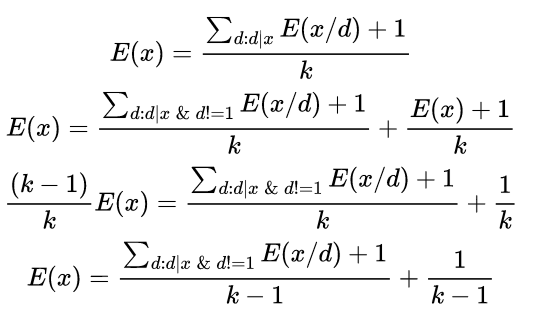We can now use math and dynamic programming to calculate E(x) through this expression.

## Approach

Calculating divisors using Harmonic Lemma

One of the subproblems is to calculate the divisors for each x from 1 to A. We can do the same using the following code

``````vector<vector<int>> divisors(A+1);
for(int i=2; i<=A; i++){
for(int mul=i; mul<=A; mul+=i){
divisors[mul].push_back(i);
}
}``````

The number of operations performed in the above code can be given as A/1 + A/2 + ... A/A which is bounded by O(AlogA).

Using Fermat's Little Theorem and Binary exponentiation to calculate Multiplicative Modular Inverse

Solving for a given x using Dynamic programming

Let dp[i] denote the expected value of step for a given i. Now we can use the expression derived earlier to calculate E(x) by dynamic programming as follows:

``````vector<int> dp(A+1,0);
for(int i=2; i<=A; i++){
dp[i] = (0LL + dp[i] + (1LL*(1)*expm(divisors[i].size(),mod-2))%mod)%mod;
for(int d:divisors[i]){
dp[i] = (0LL + dp[i] + (1LL*(dp[i/d]+1)*expm(divisors[i].size(),mod-2))%mod)%mod;
}
}``````

Note that we dont add 1 in the list of divisors hence we dont need to subtract 1 from k in the denominator.

Since each number is chosen randomly and equiprobable the final answer can be obtained by summing the value of E(x) for each x from 1 to A and then multiplying by 1/A (that is by the multiplicative inverse of A modulo the given prime).

``````int ans = 0;
for(int i=1; i<=A; i++) ans = (ans + dp[i])%mod;
ans = (1LL*ans *  expm(A,mod-2))%mod;``````

We have thus solved the problem in a complexity of O(AlogA)

1
Entering edit mode

# Problem 1 Solution

## Topics Involved / Prerequisites

• Sweep line
• Sorting
• Segment Tree / Binary Indexed Tree (Fenwick Tree) / Order Statistics Tree

## Overview

Instead of finding out the number of monsters alive after the i-th hero has appeared, lets focus on the number of monsters the i-th hero kills. Notice that a hero on some coordinate xdoes not affect the monsters at other coordinates. Hence we can focus on the heroes at each coordinate individually. Now lets consider the heroes from left to right, i.e. in increasing order of coordinates. We can maintain a data structure for the monsters at the current coordinate as we move from left to right. Now lets consider the heroes at the current coordinate in increasing order of their time of appearance.

Let the max strength of some hero considered so far at the current coordinate be x. We can have two cases:

• The strength of current hero is smaller than or equal to x, in which case it will not kill any monster (simply because other heroes would have already killed all the monsters that this hero could).
• The strength of current hero is strictly greater than x, in which case it will kill all the monsters whose strength lie in the range [x+1, strength of current hero].

We can simply query the data structure to obtain the answer for each hero in this way to solve the problem efficiently.

## Approach

Performing the Sweep line

For each monster, we will create two events - 1) + l s --- inserts a monster of strength s on coordinate l

2) - r s --- removes a monster of strength s from coordinate r

Now as we move along the coordinates we will have to first process all the events at the current coordinate and update our data structure (insert or remove some monster) before considering the heroes.

Maintaining the data structure

We require our data structure to support insert, delete and order statistics queries efficiently. We can use a coordinate compressed Segment Tree/ Fenwick Tree for the same. Note that we can not use the c++ PBDS for this because it cannot support duplicates.

We will build a segment tree over the values of the monsters and support the operations as follows:

• To insert a monster of strength s, we will increase the value at the compressed index corresponding to strength s. (point update query)
• To delete a monster of strength s, we will decrease the value at the compressed index corresponding to strength s. (point update query)
• To answer the number of elements between a range [l,r], we will perform a range sum query over the compressed indices of l and r.

Calculating the answer for each hero

Now that we are performing the sweep line and have updated our data structure at the current coordinate as explained, we sort all the heroes at the current coordinate in increasing order of time of appearance. We have to maintain the maximum strength of hero considered so far for the current coordinate and update it accordingly.

## Code

``````using ll = long long;
class segtree{
vector<ll> tree, coordinates;
public:
segtree(vector<ll>& val)
: coordinates(val)
{}

void compress(){
sort(coordinates.begin(),coordinates.end());
coordinates.erase(unique(coordinates.begin(),coordinates.end()),coordinates.end());
tree.resize(2*coordinates.size(),0);
}

void update(int value, int incr){
int indx = lower_bound(coordinates.begin(),coordinates.end(),value) - coordinates.begin();
indx += tree.size()/2;
tree[indx] += incr;
while(indx>1){
indx >>= 1;
tree[indx] = tree[2*indx] + tree[2*indx+1];
}
}

ll query(int value_l, int value_r){
int l = lower_bound(coordinates.begin(),coordinates.end(), value_l) - coordinates.begin();
int r = lower_bound(coordinates.begin(),coordinates.end(), value_r) - coordinates.begin();
l += tree.size()/2, r += tree.size()/2;
ll ans = 0;
while(r>l){
if(l&1) ans += tree[l++];
if(r&1) ans += tree[--r];
l >>= 1, r >>= 1;
}
return ans;
}
};

vector<ll> solve(vector<vector<int>>& A, vector<vector<int>>& B){
constexpr int N = 1e5 + 1;
ll total = 0;
vector<vector<pair<int,int>>> events(N);
vector<ll> strengths, ans(B.size(),0), heroes[N];
for(auto& monster:A){
total += monster - monster+1;
events[monster].push_back({1,monster});
if(monster+1<N) events[monster+1].push_back({-1,monster});
strengths.push_back(monster);
}
for(int i=0; i<B.size(); i++){
strengths.push_back(B[i]);
heroes[B[i]].push_back(i);
}
segtree tree(strengths); tree.compress();
for(int i=1; i<N; i++){
for(auto& [incr,value]:events[i]) tree.update(value,incr);
int max_strength_so_far = 0;
for(auto hero_id:heroes[i]){
if(B[hero_id] > max_strength_so_far){
ans[hero_id] = tree.query(max_strength_so_far+1,B[hero_id]);
max_strength_so_far = B[hero_id];
}
}
}
for(int i=1; i<ans.size(); i++) ans[i] += ans[i-1];
for(int i=0; i<ans.size(); i++) ans[i] = total - ans[i];
return ans;
};``````

0
Entering edit mode

Hey Ayush Gangwani, can you also tell me link of problem 1 on codeforces. please

Entering edit mode
0

The problem is live on TJO, use this link http://thejoboverflow.com/problem/373/

Entering edit mode
0

Can you help me understand the sample testcase 2 i.e. n = 2, expected output is given 1, but according to my calculation expected step is (1/4) + (1/4 ) + (1/4 ) +...., how is this converging to 1? Or correct me if I understood any part wrong.

0
Entering edit mode

I used an array of sets for this question, and it passed two test cases but failed for the limiting memory cases.Similar Posts